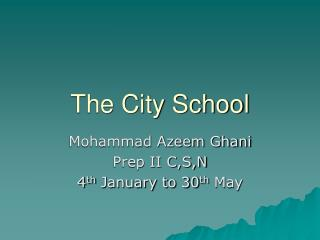DownloadDownload PresentationThe City School

# The City School

Download Presentation## The City School

- - - - - - - - - - - - - - - - - - - - - - - - - - - E N D - - - - - - - - - - - - - - - - - - - - - - - - - - -
##### Presentation Transcript

1. The City School Mohammad Azeem Ghani Prep II C,S,N 4th January to 30th May

2. Mathematics • Everyday calculation problems • Algebra • Coordinate Geometry • Statistics

3. Everyday Calculation Problem • Percentages • Ratio Proportion • Profit and Loss • Simple interest • Hire purchases • Rate conversion

4. Algebra • Simplification • Expansion • Factorization • Perfect Square Identities • Solving Equation

5. Coordinate Geometry • Coordinate Mapping • Axis Identification • Mid-point Calculation • Distance Formula • Linear Cartesian Coordinate Equation

6. Statistics • Tally Mark • Bar Chart • Mean, Median and Mode • Frequency Chart • Frequency Ploygon

7. There are lot of interesting worksheet material is available to practice MATHEMATICS I look forward to teach you student in the year ahead. So that you may prosper in future.